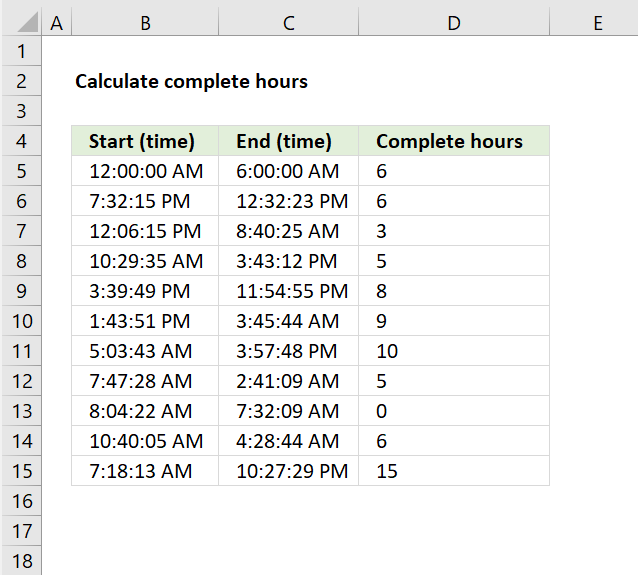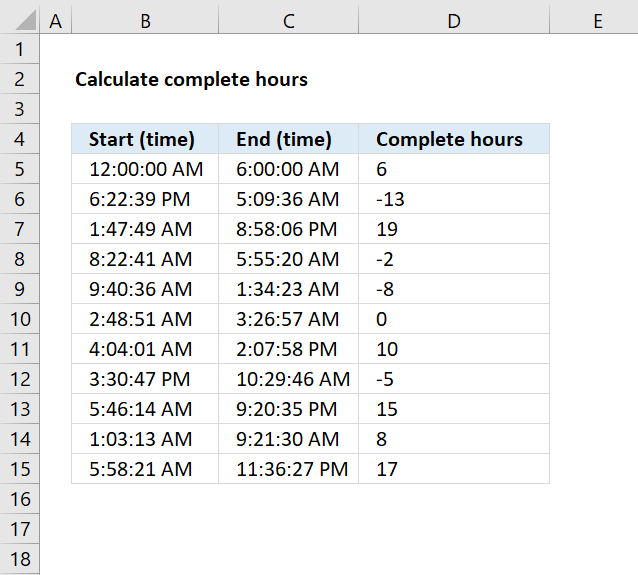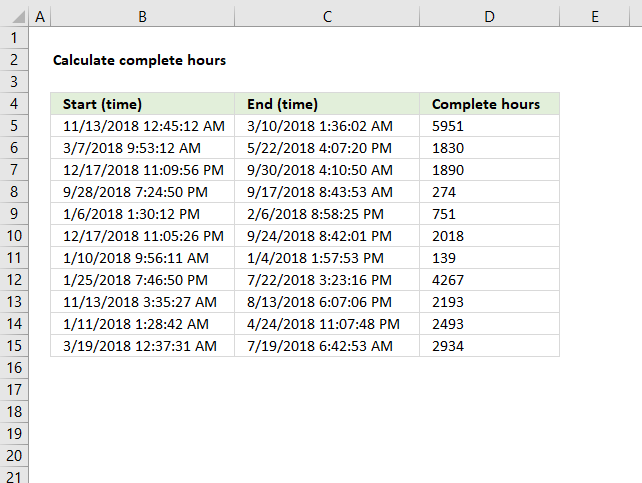Author: Oscar Cronquist Article last updated on October 24, 2018The formula in cell D5 calculates the number of complete hours between the time entries in cell B5 and C5.

Formula in cell D5:

=HOUR(ABS(C5-B5))

Note that this formula works only with time entries, not date and time entries. It doesn't matter if the start time is past the end time, the formula will regardless return a positive hour value.

### Explaining formula in cell D5

#### Step 1 - Subtract time entries

C5-B5

becomes

6:00:00 AM - 12:00:00 AM

Time entries in excel are actually a decimal value between 0 (zero) and 1. One hour is 1/24, six hours is 6/24 and so on.

0.25 - 0 equals 0.25

#### Step 2 - Remove sign

The ABS function removes the minus sign if it exists, the HOUR function can't calculate negative values.

ABS(C5-B5)

becomes

ABS(0.25) and returns 0.25.

#### Step 3 - Convert decimal to hour(s)

The HOUR function calculates hours based on a decimal value.

HOUR(ABS(C5-B5))

becomes

HOUR(0.25)

and returns 6.The image above demonstrates a formula the returns negative hours if the start time is later than the end time.

Formula in cell D5:

=HOUR(ABS(C5-B5))*IF(C5<B5,-1,1)

### Explaining formula in cell D5

This part of the formula HOUR(ABS(C5-B5)) is explained above.

#### Step 1 - Calculate if end date is smaller than the start date

C5<B5

becomes

0.25<0

and returns FALSE.

#### Step 2 - Return -1 if TRUE and 1 if FALSE

IF(C5<B5,-1,1)

becomes

IF(0.25<0,-1,1)

becomes

IF(FALSE,-1,1) and returns 1.

#### Step 3 - Multiply with result

HOUR(ABS(C5-B5))*IF(C5<B5,-1,1)

becomes

6*IF(C5<B5,-1,1)

becomes

6*1 and returns 6 in cell D5.The picture above shows a formula that calculates complete hours between date and time entries.

Formula in cell D5:

=TEXT(ABS(C5-B5),"[h]")

If you want negative hours then remove the ABS function:

=TEXT(C5-B5,"[h]")

The TEXT function formats the result of C5-B5 into complete hours.